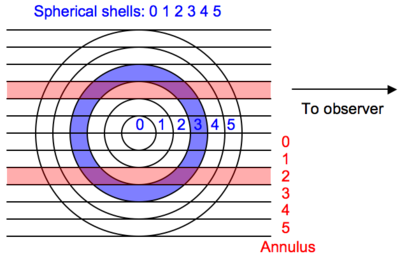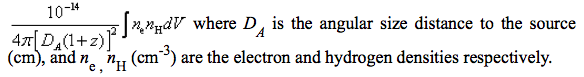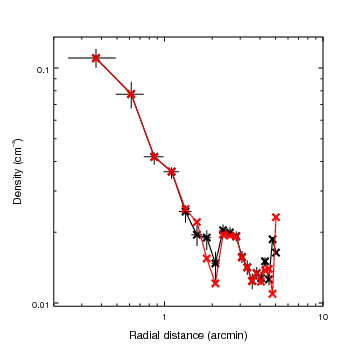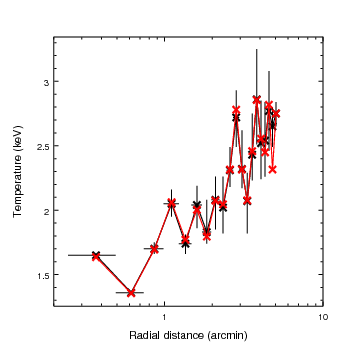# Deproject¶

`Deproject` is a CIAO Sherpa extension package to facilitate deprojection of two-dimensional annular X-ray spectra to recover the three-dimensional source properties. For typical thermal models this would include the radial temperature and density profiles. This basic method has been used extensively for X-ray cluster analysis and is the basis for the XSPEC model projct. The `deproject` module brings this functionality to Sherpa as a Python module that is straightforward to use and understand.

The `deproject` module uses `specstack` to allow for manipulation of a stack of related input datasets and their models. Most of the functions resemble ordinary Sherpa commands (e.g. set_par, set_source, ignore) but operate on a stack of spectra.

The basic physical assumption of `deproject` is that the extended source emissivity is constant and optically thin within spherical shells whose radii correspond to the annuli used to extract the specta. Given this assumption one constructs a model for each annular spectrum that is a linear volume-weighted combination of shell models. The geometry is illustrated in the figure below (which would be rotated about the line to the observer in three-dimensions):## Model creation¶

It is assumed that prior to starting `deproject` the user has extracted source and background spectra for each annulus. By convention the annulus numbering starts from the inner radius at 0 and corresponds to the dataset `id` used within Sherpa. It is not required that the annuli include the center but they must be contiguous between the inner and outer radii.

Given a spectral model `M[s]` for each shell `s`, the source model for dataset `a` (i.e. annulus `a`) is given by the sum over `s >= a` of `vol_norm[s,a] * M[s]` (normalized volume * shell model). The image above shows shell 3 in blue and annulus 2 in red. The intersection of (purple) has a physical volume defined as `vol_norm[3,2] * v_sphere` where `v_sphere` is the volume of the sphere enclosing the outer shell.

The bookkeeping required to create all the source models is handled by the `deproject` module.

## Fitting¶

Once the composite source models for each dataset are created the fit analysis can begin. Since the parameter space is typically large the usual procedure is to initally fit using the “onion-peeling” method:

• First fit the outside shell model using the outer annulus spectrum
• Freeze the model parameters for the outside shell
• Fit the next inward annulus / shell and freeze those parameters
• Repeat until all datasets have been fit and all shell parameters determined.

From this point the user may choose to do a simultanenous fit of the shell models, possibly freezing some parameters as needed. This process is made manageable with the `specstack` methods that apply normal Sherpa commands like freeze or set_par to a stack of spectral datasets.

## Densities¶

Physical densities (cm^-3) for each shell can be calculated with `deproject` assuming the source model is based on a thermal model with the “standard” normalization (from the XSPEC documentation):Inverting this equation and assuming a constant ratio of N_H to electrons:

```n_e = sqrt(norm * 4*pi * DA^2 * 1e14 * (1+z)^2 / (volume * ne_nh_ratio))

norm        = model normalization from Sherpa fit
DA          = angular size distance (cm)
volume      = volume (cm^3)
ne_nh_ratio = 1.18
```

Recall that the model components for each volume element (intersection of the annular cylinder `a` with the spherical shell `s`) are multiplied by a volume normalization:

```vol_norm[s,a] = v[s,a] / v_sphere
v_sphere = volume of sphere enclosing outer annulus
```

With this convention the `volume` used above in calculating the electron density for each shell is always `v_sphere`.

The `deproject` package is available for download at deproject.tar.gz. The M87 data needed to run the example analysis is available as m87.tar.gz

The source is available on github at https://github.com/taldcroft/deproject.

# Installation¶

The `deproject` package includes three Python modules that must be made available to the CIAO python so that Sherpa can import them. The first step is to untar the package tarball, change into the source directory, and initialize the CIAO environment:

```tar zxvf deproject-<version>.tar.gz
tar zxvf m87.tar.gz -C deproject-<version>/examples   # Needed for example / test script
cd deproject-<version>
source /PATH/TO/ciao/bin/ciao.csh
```

There are three methods for installing. Choose ONE of the three.

Simple:

The very simplest installation strategy is to just leave the module files in the source directory and set the `PYTHONPATH` environment variable to point to the source directory:

```setenv PYTHONPATH \$PWD
```

This method is fine in the short term but you always have to make sure `PYTHONPATH` is set appropriately (perhaps in your ~/.cshrc file). And if you start doing much with Python you will have `PYTHONPATH` conflicts and things will get messy.

Better:

If you cannot write into the CIAO python library then do the following. These commands create a python library in your home directory and install the `deproject` modules there. You could of course choose another directory instead of `\$HOME` as the root of your python library.

```mkdir -p \$HOME/lib/python
python setup.py install --home=\$HOME
setenv PYTHONPATH \$HOME/lib/python
```

Although you still have to set `PYTHONPATH` this method allows you to install other Python packages to the same library path. In this way you can make a local repository of packages that will run within Sherpa.

Best:

If you have write access to the CIAO installation you can just use the CIAO python to install the modules into the CIAO python library. Assuming you are in the CIAO environment do:

```python setup.py install
```

This puts the new modules straight in to the CIAO python library so that any time you enter the CIAO environment they will be available. You do NOT need to set `PYTHONPATH`.

# Test¶

To test the installation change to the source distribution directory and do the following:

```cd examples
sherpa
execfile('fit_m87.py')
plot_fit(0)
log_scale()
```

This should run through in a reasonable time and produce output indicating the onion-peeling fit. The plot should show a good fit.

# Example: M87¶

Now we step through in detail the `fit_m87.py` script in the `examples` directory to explain each step and illustrate how to use the `deproject` module. This script should serve as the template for doing your own analysis.

This example uses extracted spectra, response products, and analysis results for the Chandra observation of M87 (obsid 2707). These were kindly provided by Paul Nulsen. Results based on this observation can be found in Forman et al 2005 and via the CXC Archive Obsid 2707 Publications list.

The first step is to tell Sherpa about the Deproject class and set a couple of constants:

```from deproject import Deproject

redshift = 0.004233                     # M87 redshift
arcsec_per_pixel = 0.492                # ACIS plate scale
angdist = 4.9e25                        # M87 distance (cm) (16 Mpc)
```

Next we create a numpy array of the the annular radii in arcsec. The numpy.arange method here returns an array from 30 to 640 in steps of 30. These values were in pixels in the original spectral extraction so we convert to arcsec. (Note the convenient vector multiplication that is possible with numpy.)

```radii = numpy.arange(30., 640., 30) * arcsec_per_pixel
```

The `radii` parameter must be a list of values that starts with the inner radius of the inner annulus and includes each radius up through the outer radius of the outer annulus. Thus the `radii` list will be one element longer than the number of annuli.

Now the key step of creating the `Deproject` object `dep`. This object is the interface to the all the `deproject` methods used for the deprojection analysis.

```dep = Deproject(radii, theta=75, angdist=angdist)
```

If you are not familiar with object oriented programming, the `dep` object is just a thingy that stores all the information about the deprojection analysis (e.g. the source redshift, PHA file information and the source model definitions) as object attributes. It also has object methods (i.e. functions) you can call such as `dep.get_par(parname)` or `dep.load_pha(file)`. The full list of attributes and methods are in the `deproject` module documentation.

In this particular analysis the spectra were extracted from a 75 degree sector of the annuli, hence `theta=75` in the object initialization. For the default case of full 360 degree annuli this is not needed. Because the redshift is not a good distance estimator for M87 we also explicitly set the angular size distance.

Now load the PHA spectral files for each annulus using the Python `range` function to loop over a sequence ranging from 0 to the last annulus. The `load_pha()` call is the first example of a `deproject` method (i.e. function) that mimics a Sherpa function with the same name. In this case `dep.load_pha(file, annulus)` loads the PHA file using the Sherpa load_pha function but also registers the dataset in the spectral stack:

```for annulus in range(len(radii)-1):
```

The `annulus` parameter is required in `dep.load_pha()` to support analysis of multi-obsid datasets.

With the data loaded we set the source model for each of the spherical shells with the `set_source()` method. This is one of the more complex bits of `deproject`. It automatically generates all the model components for each shell and then assigns volume-weighted linear combinations of those components as the source model for each of the annulus spectral datasets:

```dep.set_source('xswabs * xsmekal')
```

The model expression can be any valid Sherpa model expression with the following caveats:

• Only the generic model type should be specified in the expression. In typical Sherpa usage one generates the model component name in the model expression, e.g. `set_source("xswabs.abs1 * xsmekal.mek1")`. This would create model components named `abs1` and `mek1`. In `dep.set_source()` the model component names are auto-generated as `<model_type>_<shell>`.
• Only one of each model type can be used in the model expression. A source model expression like “xsmekal + gauss1d + gauss1d” would result in an error due to the model component auto-naming.

Now the energy range used in the fitting is restricted using the stack version of the Sherpa ignore command. The notice command is also available.

```dep.ignore(None, 0.5)
dep.ignore(1.8, 2.2)
dep.ignore(7, None)
```

Next any required parameter values are set and their freeze or thaw status are set.

```dep.set_par('xswabs.nh', 0.0255)
dep.freeze("xswabs.nh")

dep.set_par('xsmekal.abundanc', 0.5)
dep.thaw('xsmekal.abundanc')

dep.set_par('xsmekal.redshift', redshift)
```

As a convenience if any of the model components have a `redshift` parameter that value will be used as the default redshift for calculating the angular size distance.

At this point the model is completely set up and we are ready to do the initial “onion-peeling” fit. As for normal high-signal fitting with binned spectra we issue the commands to set the optimization method, set the fit statistic, and configure Sherpa to subtract the background when doing model fitting. Finally the `deproject` `fit()` method is called to perform the fit.

```set_method("levmar")                    # Levenberg-Marquardt optimization method
set_stat("chi2gehrels")                 # Gehrels Chi^2 fit statistic
dep.subtract()
dep.fit()
```

After the fit process each shell model has an association normalization that can be used to calculate the densities. This is where the source angular diameter distance is used. If the angular diameter distance is not set explicitly in the original `dep = Deproject(...)` command then it is calculated automatically from the redshift found as a source model parameter. One can examine the values being used as follows:

```print "z=%.5f angdist=%.2e cm" % (dep.redshift, dep.angdist)
```

The electron density is then calculated with the `get_density()` method and plotted in Sherpa:

```density_ne = dep.get_density()
set_curve(['symbol.color', 'red', 'line.color', 'red'])
set_plot_ylabel('Density (cm^{-3})')
limits(X_AXIS, 0.2, 10)
log_scale()
print_window('m87_density', ['format', 'png'])
```

The temperature profile from the `deproject` can be plotted as follows:

```kt = dep.get_par('xsmekal.kt')   # returns array of kT values
set_plot_ylabel('Density (cm^{-3})')
```

The unphysical temperature oscillations seen here highlights a known issue with this analysis method.

In the images below the `deproject` results (red) are compared with values (black) from an independent onion-peeling analysis by P. Nulsen using a custom perl script to generate XSPEC model definition and fit commands. These plots were created with the `plot_m87.py` script in the `examples` directory. The agreement is good:# Example: Multi-obsid¶

A second example illustrates the use of `deproject` for a multi-obsid observation of 3c186. It also shows how to set a background model for fitting with the `cstat` statistic. The extracted spectral data for this example are not yet publicly available.

The script starts with some setup:

```import deproject

set_method("levmar")
set_stat("cstat")
```

Now we read in the data as before with `dep.load_pha()`. The only difference here is an additional loop over the obsids. The `dep.load_pha()` function automatically extracts the obsid from the file header. This is used later in the case of setting a background model.

```obsids = (9407, 9774, 9775, 9408)
for obsid in obsids:
```

Create and configure the source model expression as usual:

```dep.set_source('xsphabs*xsapec')
dep.ignore(None, 0.5)
dep.ignore(7, None)
dep.freeze("xsphabs.nh")

dep.set_par('xsapec.redshift', 1.06)
dep.set_par('xsphabs.nh', 0.0564)
```

Set the background model:

```execfile("acis-s-bkg.py")
acis_s_bkg = get_bkg_source()
dep.set_bkg_model(acis_s_bkg)
```

Fit the projection model:

```dep.fit()
```

# To Do¶

• Use the Python logging module to produce output and allow for a verbosity setting. [Easy]
• Create and use more generalized `ModelStack` and `DataStack` classes to allow for general mixing models. [Hard]